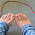# Question Bank

Question Banks of Data Structure - Set 1

Q.1 What is a data structure?
Q.2 What does abstract data type means?
Q.3 Explain about the types of linked lists.
Q.4 Write an algorithm to merge two sorted arrays into a third array.

Q.5 Write the programs for Linked List (Insertion and Deletion) operations.
Q.6 What data structure would you mostly likely see in a non recursive implementation of a recursive algorithm?
Q.7 List out the areas in which data structures are applied extensively?
Q.8 If you are using C language to implement the heterogeneous linked list, what pointer type will you use?
Q.9 What is the data structures used to perform recursion?
Q.10 What are the disadvantages array implementations of linked list?
Q.11 Whether Linked List is linear or Non-linear data structure?
Q.12 Explain what the effect will be if both continuous linked versions of sequential search have only one item in the list and when the list is empty.
Q.13 A two dimensional array TABLE   is stored in row major order with base address 351. What is the address of TABLE  ?
Q.14 What is the difference between a grounded header link list and a circular header link list?
Q.15 What values are automatically assigned to those array elements which are not explicitly initialized?
Q.16 Explain the method to calculate the address of an element in an array.
Q.17 How do you find the complexity of an algorithm? What is the relation between the time and space complexities of an algorithm?
Q.18 Compare two functions n2 and 2 4 n for various values of n. Determine when second becomes larger than first.
Q.19 Explain an efficient way of storing a sparse matrix in memory. Write a module to find the transpose of a sparse matrix stored in this way.
Q.20 Explain an efficient way of storing two symmetric matrices of the same order in memory.
Q.21 What is the difference between a Stack and an Array?
Q.22 Two linked lists contain information of the same type in ascending order.

Q.23  Define the term array. How are two-dimensional arrays represented in memory?
Q. 24 Explain how address of an element is calculated in a two dimensional array.
Q. 25 Write a module to merge them to a single linked list that is sorted.

Thanks
Mukesh RajputMukesh Rajput

I am a Computer Engineer, a small amount of the programming tips as it’s my hobby, I love to travel and meet people so little about travel, a fashion lover and love to eat food, I am investing a good time to keep the body fit so little about fitness also..

1.